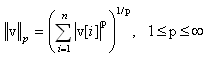# Package Modelica.​ComplexMath.​VectorsLibrary of functions operating on complex vectors

### Information

This library provides functions operating on vectors of Complex numbers.

Extends from `Modelica.​Icons.​FunctionsPackage` (Icon for packages containing functions).

### Package Contents

NameDescription
`length`Return length of a complex vector
`norm`Returns the p-norm of a complex vector
`normalize`Return normalized complex vector such that length = 1 and prevent zero-division for zero vector
`reverse`Reverse vector elements (e.g., v becomes last element)
`sort`Sort elements of complex vector

## Function Modelica.​ComplexMath.​Vectors.​normReturns the p-norm of a complex vector

### Information

#### Syntax

```Vectors.norm(v);
Vectors.norm(v,p=2);   // 1 ≤ p ≤ ∞
```

#### Description

The function call "`Vectors.norm(v)`" returns the Euclidean norm "`sqrt(v*v)`" of vector v. With the optional second argument "p", any other p-norm can be computed:Besides the Euclidean norm (p=2), also the 1-norm and the infinity-norm are sometimes used:

 1-norm = sum(abs(v)) norm(v,1) 2-norm = sqrt(v*v) norm(v) or norm(v,2) infinity-norm = max(abs(v)) norm(v,Modelica.Constants.inf)

Note, for any vector norm the following inequality holds:

```norm(v1+v2,p) ≤ norm(v1,p) + norm(v2,p)
```

#### Example

```  v = {2, -4, -2, -1};
norm(v,1);    // = 9
norm(v,2);    // = 5
norm(v);      // = 5
norm(v,10.5); // = 4.00052597412635
norm(v,Modelica.Constants.inf);  // = 4
```

Extends from `Modelica.​Icons.​Function` (Icon for functions).

### Inputs

TypeNameDescription
`Complex``v[:]`Vector
`Real``p`Type of p-norm (often used: 1, 2, or Modelica.Constants.inf)

### Outputs

TypeNameDescription
`Real``result`p-norm of vector v

## Function Modelica.​ComplexMath.​Vectors.​lengthReturn length of a complex vector

### Information

#### Syntax

```Vectors.length(v);
```

#### Description

The function call "`Vectors.length(v)`" returns the Euclidean length "`sqrt(v*v)`" of vector v. The function call is equivalent to Vectors.norm(v). The advantage of length(v) over norm(v)"is that function length(..) is implemented in one statement and therefore the function is usually automatically inlined. Further symbolic processing is therefore possible, which is not the case with function norm(..).

#### Example

```  v = {2, -4, -2, -1};
length(v);  // = 5
```

Extends from `Modelica.​Icons.​Function` (Icon for functions).

### Inputs

TypeNameDescription
`Complex``v[:]`Vector

### Outputs

TypeNameDescription
`Real``result`Length of vector v

## Function Modelica.​ComplexMath.​Vectors.​normalizeReturn normalized complex vector such that length = 1 and prevent zero-division for zero vector

### Information

#### Syntax

```Vectors.normalize(v);
Vectors.normalize(v,eps=100*Modelica.Constants.eps);
```

#### Description

The function call "`Vectors.normalize(v)`" returns the unit vector "`v/length(v)`" of vector v. If length(v) is close to zero (more precisely, if length(v) < eps), v is returned in order to avoid a division by zero. For many applications this is useful, because often the unit vector e = v/length(v) is used to compute a vector x*e, where the scalar x is in the order of length(v), i.e., x*e is small, when length(v) is small and then it is fine to replace e by v to avoid a division by zero.

Since the function is implemented in one statement, it is usually inlined and therefore symbolic processing is possible.

#### Example

```  normalize({1,2,3});  // = {0.267, 0.534, 0.802}
normalize({0,0,0});  // = {0,0,0}
```

Extends from `Modelica.​Icons.​Function` (Icon for functions).

### Inputs

TypeNameDescription
`Complex``v[:]`Vector
`Real``eps`if |v| < eps then result = v

### Outputs

TypeNameDescription
`Complex``result[size(v, 1)]`Input vector v normalized to length=1

## Function Modelica.​ComplexMath.​Vectors.​reverseReverse vector elements (e.g., v becomes last element)

### Information

#### Syntax

`Vectors.reverse(v);`

#### Description

The function call "`Vectors.reverse(v)`" returns the complex vector elements in reverse order.

#### Example

`  reverse({1,2,3,4});  // = {4,3,2,1}`

Extends from `Modelica.​Icons.​Function` (Icon for functions).

### Inputs

TypeNameDescription
`Complex``v[:]`Vector

### Outputs

TypeNameDescription
`Complex``result[size(v, 1)]`Elements of vector v in reversed order

## Function Modelica.​ComplexMath.​Vectors.​sortSort elements of complex vector

### Information

#### Syntax

```           sorted_v = Vectors.sort(v);
(sorted_v, indices) = Vectors.sort(v, ascending=true);
```

#### Description

Function sort(..) sorts a Real vector v in ascending order and returns the result in sorted_v. If the optional argument "ascending" is false, the vector is sorted in descending order. In the optional second output argument the indices of the sorted vector with respect to the original vector are given, such that sorted_v = v[indices].

#### Example

```  (v2, i2) := Vectors.sort({-1, 8, 3, 6, 2});
-> v2 = {-1, 2, 3, 6, 8}
i2 = {1, 5, 3, 4, 2}
```

Extends from `Modelica.​Icons.​Function` (Icon for functions).

### Inputs

TypeNameDescription
`Complex``v[:]`Vector to be sorted
`Boolean``ascending`= true if ascending order, otherwise descending order
`Boolean``sortFrequency`= true, if sorting is first for imaginary then for real value; = false, if sorting is for absolute value

### Outputs

TypeNameDescription
`Complex``sorted_v[size(v, 1)]`Sorted vector
`Integer``indices[size(v, 1)]`sorted_v = v[indices]

Generated 2018-12-12 12:14:36 EST by MapleSim.